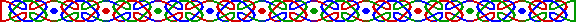(p-1)!-1 = 0 (mod p2)2p-1 = 1 (mod p2)

1092 = 100010001002

3510 = 1101101101102

pq-1 = 1 (mod q2) 及 qp-1 = 1 (mod p2)1862 年，英國數學家胡斯頓賀姆 (Joseph Wolstenholme) 證明了一個有趣的結果：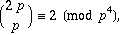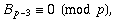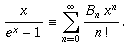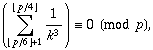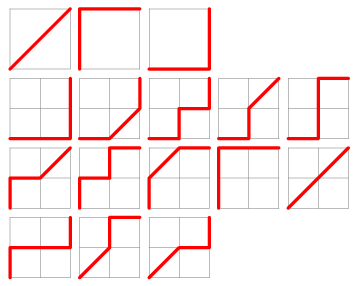D(a,b) = D(a-1,b) + D(a, b-1) + D(a-1,b-1) 其中 D(0,0) = 1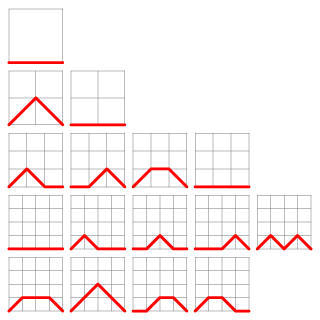Mn = (n+2)-1 * [3(n-1)Mn-2 + (2n+1)Mn-1]

Guy, R. K. §A3 in Unsolved Problems in Number Theory, 2nd ed. New York: Springer-Verlag, 1994.

Ribenboim, P. "The Little Book of Bigger Prime" , New York: Springer-Verlag, 1991

Ribenboim, P. "Wieferich Primes." §5.3 in The New Book of Prime Number Records. New York: Springer-Verlag, pp. 333-346, 1996.

Ribenboim, P. "Wilson Primes." §5.4 in The New Book of Prime Number Records. New York: Springer-Verlag, pp. 346-350, 1996.

Weisstein, E. W. "Bernoulli Number." From MathWorld. http://mathworld.wolfram.com/BernoulliNumber.html.

Weisstein, E. W. "Delannoy Number." From MathWorld. http://mathworld.wolfram.com/DelannoyNumber.html.

Weisstein, E. W. "Double Wieferich Prime Pair." From MathWorld. http://mathworld.wolfram.com/DoubleWieferichPrimePair.html.

Weisstein, E. W. "Motzkin Number." From MathWorld. http://mathworld.wolfram.com/MotzkinNumber.html.

Weisstein, E. W. "Wieferich Prime." From MathWorld. http://mathworld.wolfram.com/WieferichPrime.html.

Weisstein, E. W. "Wilson Prime." From MathWorld. http://mathworld.wolfram.com/WilsonPrime.html.

Weisstein, E. W. "Wilson Quotient." From MathWorld. http://mathworld.wolfram.com/WilsonQuotient.html.

Weisstein, E. W. "Wolstenholme Prime." From MathWorld. http://mathworld.wolfram.com/WolstenholmePrime.html.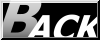Hosted by www.Geocities.ws